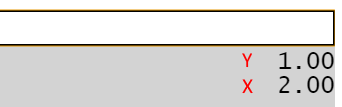# EBTCalc (Desktop) Reference

## Built-in ButtonsFigure 1: The Stack

As new value are entered, they are added to the list (stack). In the following table, if x and y are specified, x denotes the value on the bottom, and y denotes the value immediately above it. If just x is specified, x denotes the bottom value.

 Button Function Drop Removes the topmost value from the stack. Swap Exchanges the two items on the top of the stack. Clear Ent Clears the value being entered. Clear All Clears the value being entered as well as the entire stack. √x Computes the square root of x. +/- Changes the sign of x. In other words, multiplies it by -1. Fix Causes numerical values to be displayed with x digits to the right of the decimal point. Float Causes numerical values to be displayed in floating-point format. Sci Not Used to enter numbers in scientific notation. In other words, computes x * 10^y. 1 / x Computes the reciprocal of x. String Prompts the user for a string and places the string on the stack. x! Computes the factorial of x. […]→ Moves all values from an array to the stack. →[…] Moves all values on the stack into an array. x→[…] Moves the top x values on the stack into an array. Store Stores x as a named variable. Retrieve Retrieves a previously stored variable. Delete Deletes a specified variable. Clear Deletes all variables. ÷ Computes x ÷ y. × Computes x × y. − Computes x − y. + Computes x + y. y^x Computes y raised to power x. % Computes x / 100. x^2 Computes the square of x. π Computes pi. Enter Places the current value on the stack. If there is no current value, duplicates x. ← Removes the most recently entered digit or decimal point from the value in the Value Entry Area.

## Pre-Programmed Buttons

### Category: (Main)

 Button Function ⌈x⌉ Computes the ceiling of x. In other words, the smallest whole number not less than x. ⌊x⌋ Computes the floor of x. In other words, the largest whole number not greater than x. →Fraction(number, iterations) Computes an approximate fraction for x, with a precision proportional to y. For example, Find Fraction(3.14159, 100) = [3, 12, 85] since 3.14159 ≈ 3 12/85. |x| Computes the absolute value of x. 10^x Computes the inverse decimal logarithm of x. In other words, computes 10 raised to the power of x. e Computes Euler's constant. e = 2.71828... e^x Computes the inverse natural logarithm of x. Fractional Part(x) Replaces x with the fractional portion of x. Integer Part(x) Replaces x with the whole number portion of x. ln(x) Computes the natural logarithm of x. log(x) Computes the common (base 10) logarithm of x. modulo(n, divisor) Computes n modulo divisor. The modulo operation returns the integer division remainder. Round(x) Rounds x.

### Category: Dates

 Button Function Diff in Days(d1,d2) Calculates the difference, in decimal days, between two date times (Javascript Date objects). Now Calculates the current date and time (returns a Javascript Date object). String →Date Converts a string to a date/time (Javascript Date object).

### Category: Developer

 Button Function base64Decode Decodes a base64 string base64Encode Base64 encodes a string JSON Pretty Print Attractively formats a JSON string XML Pretty Print Attractively formats an XML string

### Category: Stack

 Button Function Roll ↑ Rotates stack items up. Roll ↓ Rotates stack items down.
See Stack Manipulation for further details.

### Category: Statistics

 Button Function Mean(array) Computes the average value of the values in x, which must be an array. Median(array) Computes the median value in x, which must be an array. Std. Dev.(array) Computes the population standard deviation (σ) of the values in x, which must be an array. Variance(array) Computes the population variance (σ2) of the values in x, which must be an array.

### Category: Trig

 Button Function ACos(x) Computes the inverse cosine of x, in degrees. ACosH(x) Computes the inverse hyperbolic cosine of x, in degrees. ASin(x) Computes the inverse sine of x, in degrees. ASinH(x) Computes the inverse hyperbolic sine of x, in degrees. ATan(x) Computes the inverse tangent of x, in degrees. ATanH(x) Computes the inverse hyperbolic tangent of x, in degrees. Cos(degrees) Computes the cosine of x, which must be a value in degrees. CosH(degrees) Computes the hyperbolic cosine of x, which must be a value in degrees. Degrees → Radians Converts x, which must be a value in degrees, into radians. Radians → Degrees Converts x, which must be a value in radians, into degrees. Sin(degrees) Computes the sine of x, which must be a value in degrees. SinH(degrees) Computes the hyperbolic sine of x, which must be a value in degrees. Tan(degrees) Computes the tangent of x, which must be a value in degrees. TanH(degrees) Computes the hyperbolic tangent of x, which must be a value in degrees.